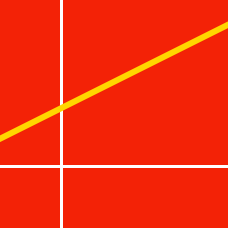Geometry

Linear Equations - Forms of a Line

The graph of the line $y=ax+b$ passes through point $(2, 90)$. If $x$ decreases from $3$ to $1$, then $y$ decreases by $4$. What is the sum of the $x$-intercept and the $y$-intercept?

What is the equation of a line that passes through two points $(-2,-2)$ and $(4, 1)$?

Let $ax-y-b=0$ be the equation of the line passing through the midpoint of the line segment connecting two points $P=(4,9)$ and $Q=(5,1)$. If the line has slope $6$, what is the value of $a+b$?

Suppose $A$ and $B$ are points in the first quadrant such that the slopes of the line segments $\overline{OA}$ and $\overline{OB}$ are $3$ and $\displaystyle \frac{1}{3}$, respectively, where $O$ is the origin. If $\lvert \overline{OA} \rvert= \lvert \overline{OB} \rvert,$ then what is the equation of line which is parallel to the line segment $AB$ and passes through the point $P=(-3,7)?$

What is the equation of a line with slope $4$ and $y$-intercept $6?$

×